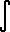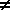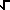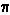Home    |    Teacher    |    Parents    |    Glossary    |    About UsEmail this page to a friendResources· Cool Tools · Formulas & Tables · References · Test Preparation · Study Tips · Wonders of MathSearchTable of Integrals (Math | Calculus | Integrals | Table Of)

Power of x.xn dx = x(n+1) / (n+1) + C  (n-1)  Proof1/x dx = ln|x| + C

Exponential / Logarithmicex dx = ex + C   Proofbx dx = bx / ln(b) + C   Proof, Tip!ln(x) dx = x ln(x) - x + C   Proof

Trigonometricsin x dx = -cos x + C   Proofcsc x dx = - ln|CSC x + cot x| + C   ProofCOs x dx = sin x + C   Proofsec x dx = ln|sec x + tan x| + C   Prooftan x dx = -ln|COs x| + C   Proofcot x dx = ln|sin x| + C   Proof

Trigonometric ResultCOs x dx = sin x + C    ProofCSC x cot x dx = - CSC x + C    Proofsin x dx = COs x + C    Proofsec x tan x dx = sec x + C    Proofsec2 x dx = tan x + C    Proofcsc2 x dx = - cot x + C    Proof

Inverse Trigonometricarcsin x dx = x arcsin x +(1-x2) + Carccsc x dx = x arccos x -(1-x2) + Carctan x dx = x arctan x - (1/2) ln(1+x2) + C

Inverse Trigonometric Resultdx(1 - x2) = arcsin x + Cdx  x(x2 - 1) = arcsec|x| + Cdx  1 + x2 = arctan x + C

 Useful Identities arccos x =/2 - arcsin x  (-1 <= x <= 1)  arccsc x =/2 - arcsec x  (|x| >= 1)  arccot x =/2 - arctan x  (for all x)

Hyperbolicsinh x dx = cosh x + C    Proofcsch x dx = ln |tanh(x/2)| + C    Proofcosh x dx = sinh x + C    Proofsech x dx = arctan (sinh x) + Ctanh x dx = ln (cosh x) + C    Proofcoth x dx = ln |sinh x| + C   Proof

Click on Proof for a proof/discussion of a theorem.

 To solve a more complicated integral, see The Integrator at http://integrals.wolfram.com/